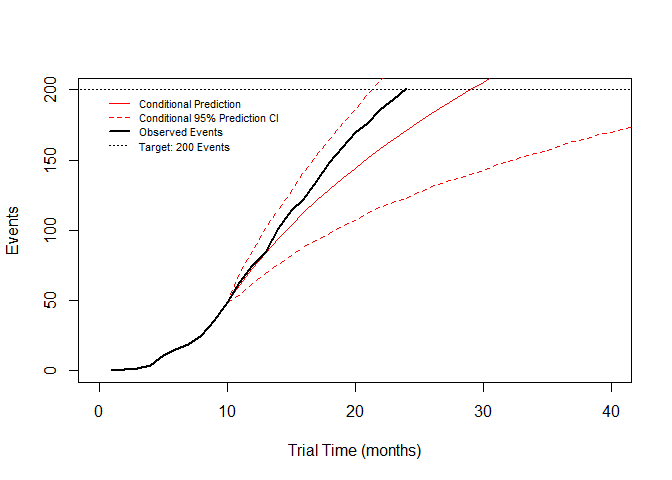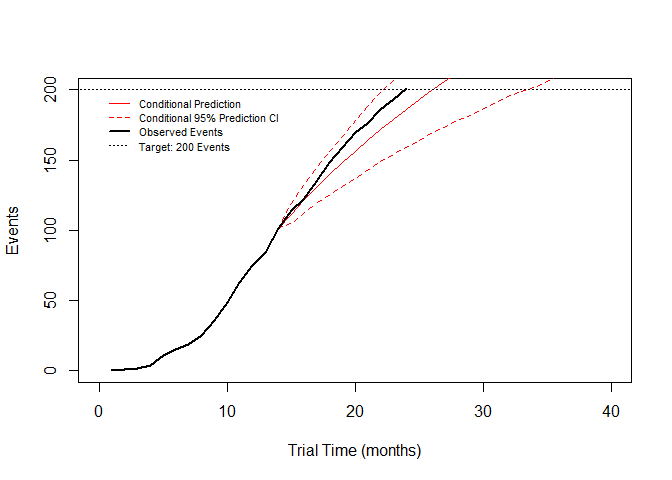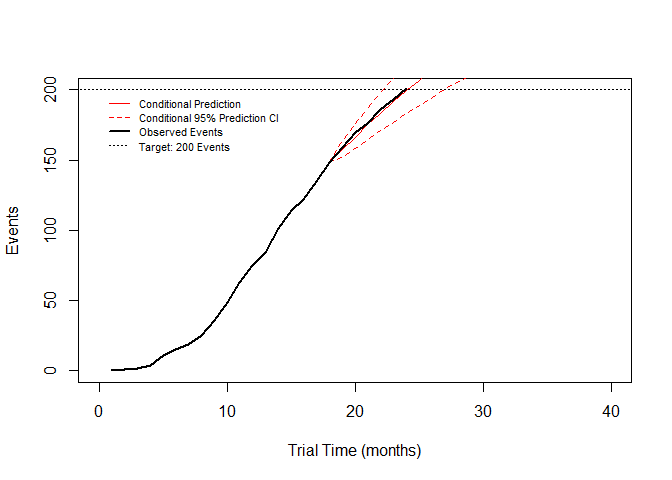# Event Prediction with the gestate Package

## 1 Introduction

### 1.1 Vignette Overview

The gestate package is designed to assist accurate planning and event prediction for time-to-event clinical trials. In this vignette, we introduce event prediction in the gestate package. A separate vignette (“trial_planning”) introduces gestate and its trial planning tools.

Section 2 will look at the two available methods for fitting parametric curves to existing data. Section 3 will then look at event prediction using these techniques.

### 1.2 Event Prediction

Event prediction is an important aspect of trial conduct for time-to-event trials since analysis time is ultimately linked to the number of events occurring, which is in turn strongly linked to power. In cases where the analysis is directly linked to event numbers, the link is obvious, but even in trials where there is a fixed assessment time, overestimation of event numbers can lead to a severe loss of power and in these cases there will often be pressure to extend trial length. It is therefore very common to either want to know the number of events that will occur at a given time, or the time at which a given number of events will occur.

### 1.3 Overview of gestate’s scope

THe gestate package supports event prediction based upon either patient-level data, or summarised life-table data. It performs parametric modelling using either Weibull or Log-normal distributions, and in cases where the approximate distribution is not known, can automatically choose the best fit. As it is based upon Curve architecture, it also supports arbitrary censoring and recruitment distributions, allowing for flexibility of assumptions. Both conditional and unconditional prediction are included. Where patient-level data is supplied, it will also produce analytic confidence intervals for predictions.

## 2 Curve-Fitting

Fitting parametric distributions to a Survival curve is useful for both event prediction and for gathering trial planning assumptions. The gestate package supports curve fitting for both Weibull and Log-normal distributions on both life-table data (fit_KM) patient-level data (fit_tte_data).

### 2.1 Example Data Set

In this section, we will use the following data set generated randomly (by simulate_trials) assuming an underlying Weibull distribution with parameters alpha = 100, beta = 0.8. For more details on this step, see section 4.1 in the vignette on trial planning (“trial_planning”). The second section of this code generates a 7-day interval lifetable; this is to create a data set that can be used to illustrate the use of fit_km, which operates on life-table data, and typically if you have patient-level data available you should use that directly instead.

library(gestate)
library(survival)
# Simulate a single data set based upon a Weibull curve with shape 0.8 and scale 100:
# Distributions
example_distribution <- Weibull(alpha=100,beta=0.8)
example_recruitment  <- LinearR(rlength=24,Nactive=600,Ncontrol=0)
# Create the full trajectory
maxtime <- 100
example_data_long <- simulate_trials(active_ecurve=example_distribution,control_ecurve=example_distribution,rcurve=example_recruitment,assess=maxtime,iterations=1,seed=1234567,detailed_output=TRUE)
# Shrink the trajectory to an early time point at which event prediction will occur
example_data_short <- set_assess_time(example_data_long,18)

######################
# Create life-table with 7-day 'sampling'
# Note that this is to create an example lifetable and would not normally be done as it is typically better to use patient-level data if available
temp1  <- summary(survfit(Surv(example_data_short[,"Time"],1-example_data_short[,"Censored"])~ 1,error="greenwood"))
out1 <- cbind(temp1$time,temp1$n.risk,temp1$surv,temp1$std.err)
out1 <- rbind(c(0,out1[1,2],1,0),out1)
colnames(out1) <- c("Time","NAR","Survival","Std.Err")
interval <- 1
x1 <- ceiling(max(out1[,"Time"]/interval))*interval
times1 <- seq(from = 0, to = x1,by=interval)
keys1 <- findInterval(times1,out1[,"Time"])
example_lifetable <- out1[keys1,]
example_lifetable[,"Time"] <- times1



### 2.2 Curve-Fitting Life-Tables: fit_KM

The fit_KM function performs a (weighted) non-linear regression on the Survival function from a life-table. It is meant for use in situations where patient-level data is unavailable, for instance where the only source of information is a Kaplan Meier plot from a publication.

In general it has inferior performance to fit_tte_data in all cases where both methods can be applied. Although with the use of suitable weighting it is approximately unbiased in its parameter estimation, it is not possible to analytically obtain accurate estimates of parameter estimate variability by this method. Stochastically it can however be seen to have higher variability than patient-level MLE-based methods such as fit_tte_data.

The ‘KMcurve’ argument specifies the name of the life-table, while the ‘Survival’ and ‘Time’ arguments can be used to change the names of the relevant columns. Due to the inevitable positive correlation between Survival points and the means of fitting, it works best if the input life-table has a constant interval regardless of timing of event occurrence (e.g. one point every 7 days). With an event-driven interval, it will typically be biased towards fitting parts of the dataset with the most events.

The ‘type’ argument specifies which parametric curve type to fit; ‘Weibull’,‘Lognormal’ or ‘automatic’. If automatic (the default) is chosen, both Weibull and Log normal are fitted and the one with the lowest (weighted) residual sum of squares is selected. If there are any convergence issues, the ‘startbeta’ and ‘startsigma’ parameters may be used to tweak the starting parameter values for Weibull and Log normal curves respectively.

To reflect that variability of the Survival function is strongly associated with the number of patients at risk, it is strongly recommended to weight the non-linear regression by the number at risk. Weighting is required by default, but may be turned off with the ‘weighting’ argument. The name of the weights column is specified by the ‘weights’ argument. By default, the weights are the values from the specified column, but the ‘weight_power’ argument optionally provides a power to raise them to.

Although the fitting process returns a covariance matrix for the parameter estimates, these are heavily underestimated since the regression is performed upon data points that are highly correlated (since each KM estimate can be described as the previous one multiplied by the subsequent probability of an event).

# Curve-fit the example lifetable in automatic mode
fit1 <- fit_KM(KMcurve=example_lifetable,Survival="Survival",Time="Time",Weights="NAR",type="automatic")
fit1
#> $Curvetype #>  "Weibull" #> #>$Parameters
#>      Alpha       Beta
#> 73.4954060  0.9179521
#>
#> $VCov #> Alpha_Var Beta_Var Covariance #> 90.590288609 0.003096191 -0.516469651 #> #>$Fit
#>      Distribution Metric                    Value
#> [1,] "Weibull"    "Weighted Sum of Squares" "0.391506961863065"
#> [2,] "Lognormal"  "Weighted Sum of Squares" "0.590485691670357"

### 2.3 Curve-Fitting Patient-Level Data: fit_tte_data

Maximum Likelihood Estimation (MLE) is a more efficient method than non-linear regression of the Kaplan Meier curve and also allows for reasonable quantification of errors. fit_tte_data uses the Survfit R function to MLE fit Weibull or Log normal parametric curves to the Survival function. Where patient-level data is available, it is therefore recommended to use fit_tte_data over fit_KM.

The ‘data’ argument specifies the data set, while the ‘Time’ and ‘Event’ arguments provide the column names for the times and event indicators respectively. The ‘censoringOne’ argument tells the function whether a 1 represents a censoring (TRUE) or an event (FALSE, default). As with fit_KM, the ‘type’ argument gives a choice of Weibull, Log normal or automatic fitting. In automatic mode, the selection is based on the higher log-likelihood (since both distributions have 2 parameters, this corresponds to the lower AIC). The ‘init’ argument may be used to provide starting values to Survfit; this will not work in automatic mode however.

# Curve-fit the example lifetable in automatic mode
fit2 <- fit_tte_data(data=example_data_short,Time="Time",Event="Censored",censoringOne=TRUE,type="automatic")
fit2
#> $Curvetype #>  "Weibull" #> #>$Parameters
#>      Alpha       Beta
#> 74.9044606  0.8971022
#>
#> $VCov #> Alpha_Var Beta_Var Covariance #> 427.253348 0.010806 -1.827576 #> #>$Fit
#>      Distribution Metric           Value
#> [1,] "Weibull"    "Log-Likelihood" "-300.998710542156"
#> [2,] "Lognormal"  "Log-Likelihood" "-303.185871359364"

Both fitting functions return a list with the type of Curve that has been fit, the parameters, and finally the components of the covariance matrix of the fit.

## 3 Event Prediction: event_prediction

### 3.1 Introduction

The event_prediction function uses a combination of fit_tte_data and the numerical integration approaches found in nph_traj to produce estimates for event numbers at any given time point. There are four main inputs required for event prediction: Firstly a patient-level data set from which an event Curve can be estimated (‘data’). Secondly, an RCurve reflecting the combined already-observed recruitment and predicted future recruitment (‘rcurve’). Thirdly, a Curve reflecting the dropout censoring in the trial (‘dcurve’). Finally, optionally, a set of parameters describing what has actually been observed at the interim time point, to allow for conditional predictions; these are described in section 3.5.

Particular care should be paid to time units since measured event times are typically in days, while recruitment and timeline planning are typically in months (see section 3.2 for more on time units). event_prediction assumes that all times are in units of months, except for the event times, which may be in units of days (default) or months; this is controlled by the optional ‘units’ argument.

### 3.2 Event Curve Fitting

Section 2.3 describes the MLE fitting process and the arguments required for this. All of these arguments carry over into event_prediction.

### 3.3 Recruitment Specification

The RCurve for an event prediction should have units of months and typically be a PieceR since the exact numbers of patients that have so far entered the trial in each month will be known. The program does not consider variability in future recruitment as it is expected that by the point that event prediction is performed a sizeable amount of recruitment will have already occurred, and the variability in future recruitment will be both low and subject to negative feedback (i.e. if it drops, efforts are made to increase it and vice versa).

# Create an RCurve incorporating both observed and predicted recruitment
recruit <- PieceR(matrix(c(rep(1,12),10,15,25,30,45,60,55,50,65,60,55,30),ncol=2),1)

### 3.4 Censoring Specification

The amount of dropout censoring varies wildly between trials. The endpoint Overall Survival (OS) ought to have minimal dropout that does not tangibly affect event prediction, whereas other endpoints are likely to have enough that it warrants consideration. A dropout distribution can be supplied to event_prediction via the ‘dcurve’ argument.

It is suggested to use fit_KM or fit_tte_data to curve-fit a version of the data where dropouts are handled as events, while administrative censoring and events are handled as censored. This should produce a reasonable estimate for censoring unconditional on event occurrence (assuming independent censoring).

### 3.5 Conditioning

To calculate conditional predictions, it is necessary to specify the number of observed events at a given time, as well as preferably the number of patients remaining at risk (total number intended to be recruited minus events occurred, minus dropouts). The conditional number at risk is important because if it is known that a larger-than-expected number of patients have dropped out, then it is clear this will lower the future expected rate of events (as fewer patients than expected are at risk). Conditional predictions can be enabled by the ‘condition’ argument. The conditional time is then specified by ‘cond_Time’, conditional events by ‘cond_Events’ and conditional number at risk by ‘cond_NatRisk’. If the latter is not known, the expected number at risk will be used as a default.

### 3.7 Calculations

A Curve object is created from the fitted parameters. This is combined with the recruitment RCurve and censoring Curve to produce estimates of the expected event numbers at each integer time point using the same approach as nph_traj. Conditioned trajectories are created by scaling future events by the ratio of the conditioned number at risk to the expected number at risk at the conditioning time. The scaled number of additional events is then added to the conditioned number of events. Where no conditioned number at risk is provided, gestate assumes by default that it is the expected number minus the difference between conditioned and expected event numbers at the conditioning time; i.e. that each additional observed event than expected reduces the expected number at risk by one.

The estimation errors for the parameters are also carried over into the event prediction calculation and propagated through by the delta method to enable the estimation of standard errors on both the conditional and unconditional expected trajectories. The conditional standard error is always lower at any given time, particularly close to the time of conditioning (and it is 0 at that time). The standard errors of the trajectories are then combined with the number at risk and the event numbers via a beta binomial distribution to create confidence intervals for the conditional and unconditional predicted trajectories.

The ‘CI’ argument specifies the width of confidence interval to produce, by default 0.95 (95%).

A paper on the general approach to analytic confidence intervals and the formulae for the calculations are to be published.

### 3.8 Plotting

For convenience, there is an inbuilt plotting function for event prediction output; plot_ep. This is designed to automatically read output from event_prediction, so only requires the ‘data’ argument, specifying the name of the event_prediction output. By default, it makes use of the available information, plotting the unconditional trajectory with prediction CI, as well as the conditional ones if available. The arguments ‘trajectory’ and ‘which_CI’ can be used to eliminate some or all of these lines, while prediction_fitting can be used to switch to switch to CIs of fitting rather than prediction.

To plot the observed events as well, an observed event trajectory may also be supplied through the ‘observed’ argument. This accepts either a vector of events, corresponding to the values at times 1,2,3…, or a 2-column matrix / data-frame with the first column the time points in ascending order, and the second the event numbers.

A target number of events may also be specified using the ‘target’ argument. The optional ‘max_time’ and ‘max_E’ options override the automatic plotting area dimensions, while the ‘legend_position’ and ‘no_legend’ arguments control the legend positioning and whether it is displayed at all. Standard graphical arguments are also accepted.

### 3.9 Example

We first create a new example data set with piecewise recruitment using the simulate_trials function in gestate:

# Create the full length trial showing what 'actually' happens
trial_long <- simulate_trials(active_ecurve=Weibull(50,0.8),control_ecurve=Weibull(50,0.8),rcurve=recruit,fix_events=200,iterations=1,seed=12345,detailed_output=TRUE)
# Create a shortened version with data for use in the event prediction
trial_short <- set_assess_time(data=trial_long,time=10,detailed_output = FALSE)
# Create the trajectory of events
maxtime <- max(ceiling(trial_long[,"Assess"]))
events <- rep(NA,maxtime)
for (i in 1:maxtime){
events[i] <- sum(1-set_assess_time(trial_long,i)[,"Censored"])
}

The ‘trial_short’ object contains the patient data observable at the time of event prediction (10 months). Note that a ‘1’ in the Censored column denotes a censoring, so the censoringOne argument needs to be set to TRUE, and that the unit of time is in months, so the units argument must be “Months”. For real patient data it is more usual to have units of days, which is the default.

‘recruit’ (see section 2.3) contains the observed and predicted patient recruitment as an RCurve object. ‘events’ contains the numbers of events observed at each time point (both before and after prediction) and can be used to assess performance. At the time of event prediction, 10, there are 47 observed events and the number at risk is 500-47= 453; these will be the conditioning parameters. We assume that there is no dropout censoring.

We can now perform a simple event prediction using Weibull curve type selection:

predictions <- event_prediction(data=trial_short, Event="Censored", censoringOne=TRUE, type="Weibull", rcurve=recruit,max_time=60, condition=TRUE, cond_Events=49, cond_NatRisk=451, cond_Time=10, units="Months")

We can then plot the conditional trajectory, with confidence intervals, alongside the events that were (or will be) observed at each time point:

# Plot observed events and conditional predictions
plot_ep(predictions,trajectory="conditional",which_CI="conditional",max_time=40,observed=events,target=200,max_E=200)The plot shows a reasonable agreement between the observed trajectory and the estimated conditional trajectory, which predicts 200 events would be reached by month 29. However, the 95% confidence interval is wide (particularly on the lower bound), and the CI for the time to reach 200 events is 22-55 months. In practice therefore, event prediction at this time point is not that useful for estimating a trial end date.

We can now revisit the event prediction at two later time points (14 and 18 months), and compare the prediction accuracies:

trial_less_short <- set_assess_time(data=trial_long,time=14,detailed_output = FALSE)
predictions2 <- event_prediction(data=trial_less_short, Event="Censored",censoringOne=TRUE, type="Weibull", rcurve=recruit,max_time=60, condition=TRUE,                               cond_Events=101,cond_NatRisk=399,cond_Time=14, units="Months")

trial_mature <- set_assess_time(data=trial_long,time=18,detailed_output = FALSE)
predictions3 <- event_prediction(data=trial_mature, Event="Censored",censoringOne=TRUE, type="Weibull", rcurve=recruit,max_time=60, condition=TRUE,                               cond_Events=148,cond_NatRisk=352,cond_Time=18, units="Months")Only 4 months later, the 95% CI is down to 23-34 months, with an estimate of 27 months, while by 18 months, a tight 95% CI of 23-27 months is possible around an estimate of 25 months. The tightening of the CI over time is partly due to a reduction in the number of events remaining to be predicted, but also the increasing precision with which the parameters of the parametric Kaplan Meier curve fit. As a rule of thumb, 100 observed events appear to be required to give reasonable precision of estimation of Weibull parameters, but the greater the length of extrapolation required, the more precise the estimates have to be to give useful accuracy.

The conditional confidence interval can therefore demonstrate that in this case where a target of 200 events is desired, event prediction is probably too inaccurate to be of use at 10 months, somewhat indicative at 14 months, and fairly accurate by 18 months. As the trial has ‘progressed’, the estimate of the time of finishing has dropped from 29 months to 27 months and then again to 25 months. Depending on tolerance for risk, it may be more useful to use a smaller CI e.g. 80 or 90%.

It should be noted that all of these CIs are dependent upon the underlying distribution being Weibull, and that the same distribution applies to all patients, irrespective of e.g. the time of recruitment. They also depend on the recruitment distribution being fixed, i.e. containing no variability - this may be unreasonable for predictions at a very early time point. These limitations will tend to lead to the interval being narrower than it ought. Conversely, the confidence intervals do not account for knowledge obtained from prior trials or beliefs from the trial design stage; if there are strong prior expectations aligned with the observed data then the confidence interval may be wider than it need be.